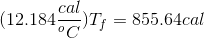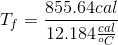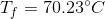# SAT II Physics : Calculating Heat and Temperature

## Example Questions

### Example Question #9 : Understanding Heat And Temperature

A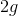disc of copper is dropped into a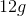glass of water. If the copper was at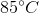and the water was at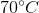, what is the new temperature of the mixture?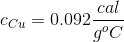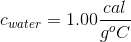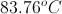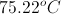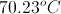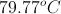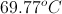Explanation:

The relationship between mass and temperature, when two masses are mixed together, is: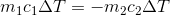Using the given values for the mass and specific heat of each compound, we can solve for the final temperature.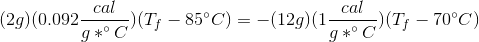We need to work to isolate the final temperature.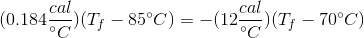Distribute into the parenthesis using multiplication.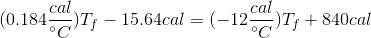Combine like terms.